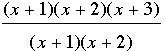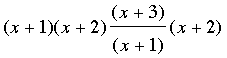SEARCH HOMEMath Central Quandaries & QueriesQuestion from Nazrul, a teacher: Simplify : (x+1)(x+2)(x+3)/(x+1)(x+2) Which answer is correct: (i) x+3 (ii) (x+2)^2(x+3) Please help me.Nazrul,

This problem annoys me. People who use mathematics try to be very precise in their use of mathematical notation. The goal is to write a mathematical expression in a way that is unambiguous so that everyone reading it has the same interpretation.

A mathematician would never write (x+1)(x+2)(x+3)/(x+1)(x+2). She would write

[(x+1)(x+2)(x+3)]/[(x+1)(x+2)]

or

(x+1)(x+2)[(x+3)/(x+1)](x+2)

ororI know there are "rules" like BEDMAS or PEDMAS which are purported to clear up any ambiguity but they are completely artificial. The correct answer is that no one who want to transmit a mathematical idea would ever write (x+1)(x+2)(x+3)/(x+1)(x+2).

HarleyMath Central is supported by the University of Regina and The Pacific Institute for the Mathematical Sciences.